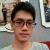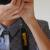php 字符串过滤/匹配 和 循环读取文件名的问题？

1:有道很简单的题目是求两个形式类似{2,6,5,8}这样的集合的交集和并集。现在我想问下，在对输入内容进行验证时 如何验证中间的元素没有出现其他字符？比如{2,*T%&,6}这样?

1.1:    我在php手册里的array_intersect函数里的注释段看到有人发表的一个小函数：

function my_array_intersect(\$a,\$b)
{
for(
\$i=0;\$i<sizeof(\$a);\$i++)
{
\$m[]=\$a[\$i];
}
for(
\$i=0;\$i<sizeof(\$b);\$i++)
{
\$m[]=\$b[\$i];
}
sort(\$m);
\$get=array();
for(
\$i=0;\$i<sizeof(\$m);\$i++)
{
if(
\$m[\$i]==\$m[\$i+1])
\$get[]=\$m[\$i];
}
return
\$get;
}

2：有个题目是在一个页面上输入日志，然后点击一个按钮提交后生成名如program201210210101.txt 的一个日志文件，并在页面上显示当前小时的日志内容。

生成日志文件倒简单，就是"program".date("YmdHi",time()).".txt" 再fopen,fwrite,fclose;

2：去掉program与".txt"并与time()-3600比较，小于则读取文件内容存到变量里输出？

如果这方法可行的话，就是第一步有点问题，(我知道stat里有filectime可以看到文件的上次修改时间，所以能不能遍历文件夹中所有的文件然后用stat得到filectime属性进行比较？)

0```function my_array_intersect( \$a, \$b ) {
for ( \$i = 0; \$i<sizeof( \$a ); \$i++ ) {
\$m[] = \$a[\$i];
}
for ( \$i = 0; \$i<sizeof( \$b ); \$i++ ) {
\$m[] = \$b[\$i];
}
sort( \$m );
\$get = array();
for ( \$i = 0; \$i<sizeof( \$m )-1; \$i++ ) {
if ( \$m[\$i]==\$m[\$i+1] ) {
\$get[] = \$m[\$i];
}
}
return array_unique(\$get);
}
var_dump(my_array_intersect( array(2,3,3,6), array(3,9,8,2) ));```

glob()函数可以扫描指定目录下的特定类型文件，相当于linux下的find命令和win的文件搜索。scandir()可以列出一个目录下的所有文件，它们都是以数组返回。

0引用来自“朱亚杰”的答案

```function my_array_intersect( \$a, \$b ) {
for ( \$i = 0; \$i<sizeof( \$a ); \$i++ ) {
\$m[] = \$a[\$i];
}
for ( \$i = 0; \$i<sizeof( \$b ); \$i++ ) {
\$m[] = \$b[\$i];
}
sort( \$m );
\$get = array();
for ( \$i = 0; \$i<sizeof( \$m )-1; \$i++ ) {
if ( \$m[\$i]==\$m[\$i+1] ) {
\$get[] = \$m[\$i];
}
}
return array_unique(\$get);
}
var_dump(my_array_intersect( array(2,3,3,6), array(3,9,8,2) ));```

glob()函数可以扫描指定目录下的特定类型文件，相当于linux下的find命令和win的文件搜索。scandir()可以列出一个目录下的所有文件，它们都是以数组返回。

1，打开页面后首先从文件目录里读取当前小时内的日志内容并存放到一变量里输出

2，用户输入日志，生成日志文件后，日志内容同时在一变量里存放。并输出